#### You may also like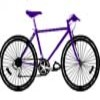### Illusion

A security camera, taking pictures each half a second, films a cyclist going by. In the film, the cyclist appears to go forward while the wheels appear to go backwards. Why?### Weighty Problem

The diagram shows a very heavy kitchen cabinet. It cannot be lifted but it can be pivoted around a corner. The task is to move it, without sliding, in a series of turns about the corners so that it is facing the other way round.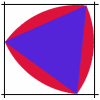### Rolling Triangle

The triangle ABC is equilateral. The arc AB has centre C, the arc BC has centre A and the arc CA has centre B. Explain how and why this shape can roll along between two parallel tracks.

# Attractive Rotations

##### Age 11 to 14Challenge Level

Attractive Rotations printable sheet
Attractive Rotations printable sheet - extension

Take a look at the image below. How do you think it was created?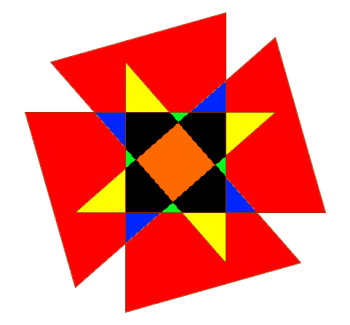Did you notice any symmetry in the image?

Here is a diagram which shows how we created the image. We started with a triangle (shaded) and then used the coordinate grid to help us to rotate it through multiples of $90^{\circ}$ around the point $(0,0)$.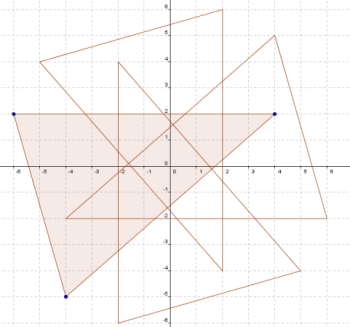Create some images of your own by rotating a shape through multiples of $90^{\circ}$.

You might like to start with a triangle as we did, or you might want to use other shapes.

How can you use a coordinate grid to help you to rotate each vertex around $(0,0)$?

What is the relationship between the coordinates of the vertices as they rotate through multiples of $90^{\circ}$?

Here are some more ideas to explore:

Can you use an isometric grid to rotate a shape through multiples of $60^{\circ}$?

Try creating some images based on other rotations, such as $30^{\circ}$ or $72^{\circ}$ or... (you will need to use a protractor for these).

Here is the kind of image you could try to create: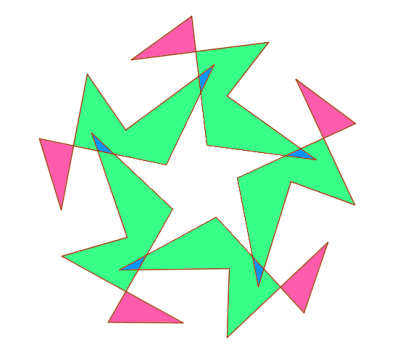What is the rotational symmetry of your final image if you rotate through multiples of $80^{\circ}$ or $135^{\circ}$? Can you explain why?

Send us pictures of your rotation patterns along with your interesting mathematical discoveries.